# HBSE 10th Class Maths Solutions Chapter 7 Coordinate Geometry Ex 7.4

Haryana State Board HBSE 10th Class Maths Solutions Chapter 7 Coordinate Geometry Ex 7.4 Textbook Exercise Questions and Answers.

## Haryana Board 10th Class Maths Solutions Chapter 7 Coordinate Geometry Ex 7.4

Question 1.
Determine the ratio in which the line 2x + y – 4 = 0 divides the line segment joining the points A(2, – 2) and B(3, 7).
Solution:
Let required ratio be k : 1 and line 2x + y – 4 divides the line segment.
Join A(2, – 2) and B(3, 7) at the point P(x, y).
Then co-ordinates of P are.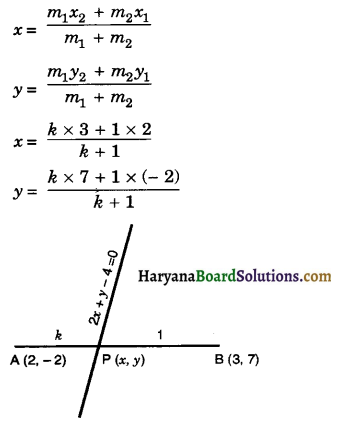⇒ x = $$\frac{3 k+2}{k+1}$$
y = $$\frac{7 k-2}{k+1}$$
Thus, the co-ordinates of P are $$\left(\frac{3 k+2}{k+1}, \frac{7 k-2}{k+1}\right)$$.
Since, point P lies on the given line 2x + y – 4 = 0.
Therefore, 2 × $$\left(\frac{3 k+2}{k+1}\right)+\frac{7 k-2}{k+1}$$ – 4 = 0
$$\frac{6 k+4+7 k-2-4 k-4}{k+1}$$ = 0
$$\frac{9 k-2}{k+1}$$ = 0
9k – 2 = 0
k = $$\frac{2}{9}$$
k : 1 = 2 : 9
Hence, required ratio is 2 : 9.Question 2.
Find a relation between x and y if the points (x, y), (1, 2) and (7, 0) are collinear.
Solution:
Let A(x, y), B(1, 2)and C(7, 0) are given points
Since, the given points are coimear.
Therefore,Area of ∆ABC = 0
⇒ $$\frac{1}{2}$$ [x1(y2 – y3) + x2(y3 – y1) + x3(y1 – y2)] = 0
⇒ $$\frac{1}{2}$$ [x(2 – 0) + 1(0 – y) + 7(y – 2)] = 0
⇒ $$\frac{1}{2}$$ [2x – y + 7y – 14] = 0
⇒ $$\frac{1}{2}$$ [2x + 6y – 14] = 0
⇒ 2x + 6y – 14 = 0
⇒ x + 3y – 7 = 0
Hence, required relation is x + 3y – 7 = 0.

Question 3.
Find the centre of a circle passing through the points (6, – 6), (3, – 7) and (3, 3).
Solution :
Let P(x, y) be the centre of a circle which passes through A(6, – 6), B(3, – 7) and C(3, 3)
∴ PA = PB = PC (equal radii of circle)
Now PA = PB
⇒ PA2 = PB2
⇒ (6 – x)2 + (- 6 – y)2 = (3 – x)2 + (- 7 – y)2
⇒ 36 + x2 – 12x + 36 + y2 + 12y = 9 + x2 – 6x + 49 + y2 + 14y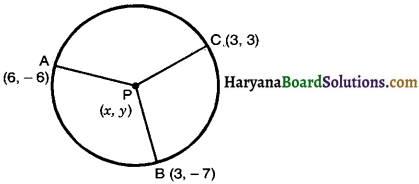⇒ x2 + y2 – 12x + 12y + 72 = x2 + y2 – 6x + 14y + 58
⇒ x2 + y2 – 12x + 12y – x2 – y2 + 6x – 14y = 58 – 72
⇒ – 6x – 2y = – 14
⇒ 3x + y = 7 ……………..(1)
and PB = PC
⇒ PB2 = PC2
⇒ (3 – x)2 + (- 7 – y)2 = (3 – x)2 + (3 – y)2
⇒ (3 – x)2 + (- 7 – y)2 – (3 – x)2 = (3 – y)2
⇒ (- 7 – y)2 = (3 – y)2
⇒ 49 + y2 + 14y = 9 + y2 – 6y
⇒ y2 + 14y – y2 + 6y = 9 – 49
20y = – 40
y = – $$\frac{40}{20}$$
y = – 2
Substituting the value ofy in equation (1), we get
3x – 2 = 7
3x = 7 + 2 = 9
x = $$\frac{9}{3}$$
x = 3
Hence, centre of the circle is (3, – 2).Question 4.
The two opposite vertices of a square are (- 1, 2) and (3, 2). Find the co-ordinates of the other two vertices.
Solution :
Let the opposite vertices of a square be A(- 1, 2) and C(3, 2) and unknown vertex be B(x, y) then
AB = BC (sides of square)
⇒ AB2 = BC2
⇒ (x + 1)2 + (y – 2)2 = (3 – x)2 + (2 – y)2
⇒ x2 + 1 + 2x + y2 + 4 – 4y = 9 + x2 – 6x + 4 + y2 – 4y
⇒ x2 + 2x – 4y + y2 + 5 = x2 + y2 – 6x – 4y + 13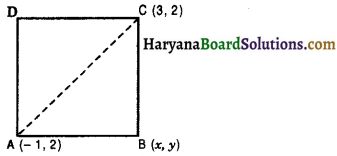x2 + 2x – 4y + y2 – y2 + 6x + 4y = 13 – 5
8x = 8
x = $$\frac{8}{8}$$
x = 1 ……………….(1)
In right ∆ABC, we get
AC2 = AB2 + BC2 [By Pythagoras theorem]
⇒ (3 + 1)2 + (2 – 2)2 = (x + 1)2 + (y – 2)2 + (3 – x)2 + (2 – y)2
⇒ 16 = x2 + 1 + 2x + y2 + 4 – 4y + 9 + x2 – 6x + 4 + y2 – 4y
⇒ 16 = 2x2 + 2y2 – 4x – 8y + 18
⇒ 2x2 + 2y2 – 4x – 8y = 16 – 18 = – 2
⇒ x2 + y2 – 2x – 4y= – 1
⇒ (1)2 + y2 – 2 × 1 – 4y = – 1 [∵ From (1) x = 1]
⇒ 1 + y2 – 4y – 2 = – 1
y2 – 4y = – 1 – 1 + 2
⇒ y(y – 4) = 0
y= 0 or y -4 = 0
y = 0 or y = 4
Hence, required vertices of the square are (1, 0) and (1, 4).Question 5.
The class X students of a secondary school in Krishinagar have been alloted a rectangular plot of land for their gardening activity. Sapling of Gulmohar are planted on the boundary at a distance of 1m from each other. There is a triangular grassy lawn in the plot as shown in the figure. The students are to sow seeds of flowering plants on the remaininq area of the plot.
(i) Taking A as origin, find the co-ordinates of the vertices of the triangle.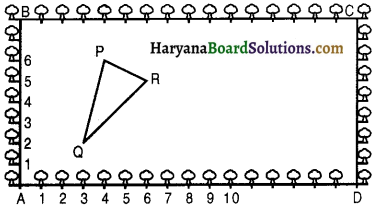(ii) What will be the coordinates of the vertices of ∆ PQR if C is the origin ? Also, calculate the area-s of the triangles in these cases. What do you observe?
Solution :
(i) Taking A as origin the coordinates of the vertices of P, Q and R are (4, 6), (3, 2) and (6, 5) respectively.
(ii) Taking C as the origin, co-ordinates of P, Q and R are (12, 2), (13, 6) and (10, 3).
In 1st condition area of ∆PQR = $$\frac{1}{2}$$ [x1(y2 – y3) + x2(y3 – y1) + x3(y1 – y2)]
= $$\frac{1}{2}$$ [4(2 – 5) + 3(5 – 6) + 6(6 – 2)]
= $$\frac{1}{2}$$ [4 × (- 3) + 3 × (- 1) + 6 × 4]
= $$\frac{1}{2}$$ (- 12 – 3 + 24)
= $$\frac{1}{2}$$ × 9
= $$\frac{9}{2}$$ square units.

In 2nd condition area of ∆PQR = $$\frac{1}{2}$$ [x1(y2 – y3) + x2(y3 – y1) + x3(y1 – y2)]
= $$\frac{1}{2}$$ [12(6 – 3) + 13(3 – 2) + 10(2 – 6)]
= $$\frac{1}{2}$$ [12 × 3 + 13 × 1 + 10 × (- 4)]
= $$\frac{1}{2}$$ (36 + 13 – 40)
= $$\frac{1}{2}$$ × 9
= $$\frac{9}{2}$$ square units
Hence, coordinates of ∆PQR.
(i) Taking A as origin and taking AD as x-axis, AB as y-axis are (4, 6), (3, 2) and (6, 5).
(ii) Taking C as origin and taking CB x-axis, CD as y-axes are (12, 2), (13, 6) and (10, 3)
Areas in two cases are $$\frac{9}{2}$$ square units.Question 6.
The vertices of a ∆ABC are A(4, 6), B(1, 5) and C(7, 2). A line is drawn to intersect sides AB and AC at D and E respectively, such that $$\frac{\mathrm{AD}}{\mathrm{AB}}=\frac{\mathrm{AE}}{\mathrm{AC}}=\frac{1}{4}$$. Calculate the area of the ∆ADE and compare it with the area of ∆ABC. (Recall theorem 6.2 and theorem 6.6).
Solution:
We have,
$$\frac{\mathrm{AD}}{\mathrm{AB}}=\frac{\mathrm{AE}}{\mathrm{AC}}=\frac{1}{4}$$
⇒ $$\frac{\mathrm{AB}}{\mathrm{AD}}=\frac{\mathrm{AC}}{\mathrm{AE}}=4$$
⇒ $$\frac{\mathrm{AB}}{\mathrm{AD}}-1=\frac{\mathrm{AC}}{\mathrm{AE}}-1$$ = 4- 1
⇒ $$\frac{\mathrm{AB}-\mathrm{AD}}{\mathrm{AD}}=\frac{\mathrm{AC}-\mathrm{AE}}{\mathrm{AE}}$$ = 3
⇒ $$\frac{\mathrm{DB}}{\mathrm{AD}}=\frac{\mathrm{EC}}{\mathrm{AE}}$$ = 3
⇒ $$\frac{\mathrm{AD}}{\mathrm{DB}}=\frac{\mathrm{AE}}{\mathrm{EC}}=\frac{1}{3}$$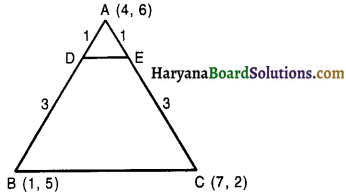⇒ AD : DB = AE : EC = 1 : 3.

Point D divides the AB in the ratio 1 : 3.
Co-ordinates of D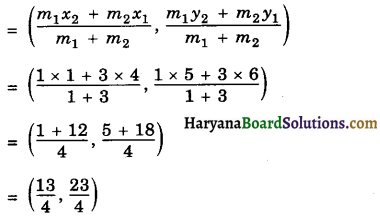Point E divides the AC in the ratio 1 : 3.
Co-ordinates of E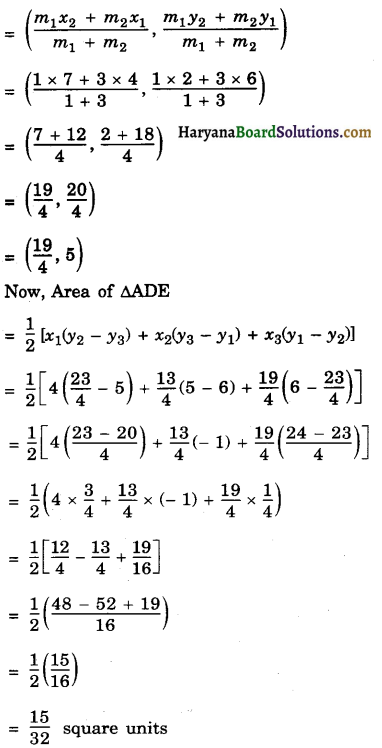Area of ∆ABC
= $$\frac{1}{2}$$ [x1 (y2 – y3) + x2(y3 – y1) + x3(y1 – y2)]
= $$\frac{1}{2}$$ [4(5 – 2) + 1(2 – 6) + 7(6 – 5)]
= $$\frac{1}{2}$$ (4 × 3 + 1 × – 4 + 7 × 1)
= $$\frac{1}{2}$$ (12 – 4 + 7)
= $$\frac{1}{2}$$ × 15
= $$\frac{15}{2}$$ square units
$$\frac{\text { Area of } \triangle \mathrm{ADE}}{\text { Area of } \triangle \mathrm{ABC}}=\frac{\frac{15}{32}}{\frac{15}{2}}$$
$$\frac{\text { Area of } \triangle \mathrm{ADE}}{\text { Area of } \triangle \mathrm{ABC}}=\frac{15}{32} \times \frac{2}{15}=\frac{1}{16}$$
Hence, Area of ∆ADE = $$\frac{15}{32}$$ square units
and Area of ADE : Area of ∆ABC = 1 : 16.Question 7.
Let A(4, 2), B(6, 5) and C(1, 4) be the vertices of ∆ABC.
(i) The median from A meets BC at D. Find the co-ordinates of the point D.
(ii) Find the co-ordinates of the points P on AD such that AP : PD = 2 : 1.
(iii) Find the co-ordinates of points Q and R on medians BE and CF respectively such that BQ : QE = 2 : 1 and CR : RF = 2 : 1.
(iv) What do you observe ?
[Note : The point which is common to all the three medians is called the centroid and this point divides each median in the ratio 2 : 1.]
(v) If A(x1, y1), B(x2, y2) and C(x3, y3) are the vertices of ∆ABC, find the co-ordinates of the centroid of the triangle.
Solution :
(i) D is the mid-point of BC.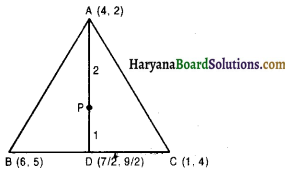Co-ordinates of point D are $$\left(\frac{6+1}{2}, \frac{5+4}{2}\right)$$ i.e., $$\left(\frac{7}{2}, \frac{9}{2}\right)$$.

(ii) P divides the AD in the ratio 2 : 1.
Co-ordinates of point P = $$\left(\frac{m_1 x_2+m_2 x_1}{m_1+m_2}, \frac{m_1 y_2+m_2 y_1}{m_1+m_2}\right)$$
= $$\left(\frac{2 \times \frac{7}{2}+1 \times 4}{2+1}, \frac{2 \times \frac{9}{2}+1 \times 2}{2+1}\right)$$
= $$\left(\frac{7+4}{3}, \frac{9+2}{3}\right)=\left(\frac{11}{3}, \frac{11}{3}\right)$$

(iii) E is the mid-point of AC.
Co-ordinates of point E = $$\left(\frac{4+1}{2}, \frac{2+4}{2}\right)=\left(\frac{5}{2}, 3\right)$$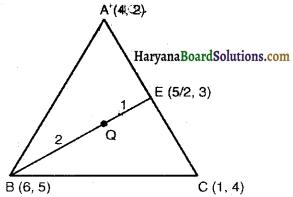Q divides BE in the.ratio 2 : 1.
Co-ordinates of point Q.
= $$\left(\frac{m_1 x_2+m_2 x_1}{m_1+m_2}, \frac{m_1^{\prime} y_2+m_2 y_1}{m_1+m_2}\right)$$
= $$\left(\frac{2 \times \frac{5}{2}+1 \times 6}{2+1}, \frac{2 \times 3+1 \times 5}{2+1}\right)$$
= $$\left(\frac{5+6}{3}, \frac{6+5}{3}\right)=\left(\frac{11}{3}, \frac{11}{3}\right)$$
F is the mid-point of AB.
Co-ordinates of point F = $$\left(\frac{4+6}{2}, \frac{2+5}{2}\right)=\left(5, \frac{7}{2}\right)$$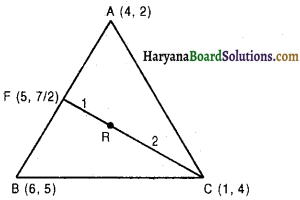Point R divides the CF in the ratio 2 : 1.
Co-ordinates of point R = $$\left(\frac{m_1 x_2+m_2 x_1}{m_1+m_2}, \frac{m_1 y_2+m_2 y_1}{m_1+m_2}\right)$$
= $$\left(\frac{2 \times 5+1 \times 1}{2+1}, \frac{2 \times \frac{7}{2}+1 \times 4}{2+1}\right)$$
= $$\left(\frac{10+1}{3}, \frac{7+4}{3}\right)=\left(\frac{11}{3}, \frac{11}{3}\right)$$

(iv) We observe that the co-ordinates of point P, Q, R, are same. Therefore, the point P, Q, R, are same point.
(v) See solution in Basic concepts; co-ordinates of the centroid.

Question 8.
ABCD is a rectangle formed by the points A(- 1, – 1), B(- 1, 4), C(5, 4) and D(5, – 1). P, Q, R and S are the mid points of AB, BC, CD and DA respectively. Is the quadrilateral PQRS a square ? a rectangle ? or a rhombus ? Justify your answer.
Solution :
Since P, Q, R and S are the mid points of the sides AB, BC, CD and DA respectively.
∴ Co-ordinates of point P are $$\left[\frac{(-1-1)}{2}, \frac{(-1+4)}{2}\right]$$ i.e., (- 1, $$\frac{3}{2}$$)
Co-ordinates of point Q are $$\left[\left(\frac{-1+5}{2}\right),\left(\frac{4+4}{2}\right)\right]$$ i.e., (2, 4)
Co-ordinates of point R are $$\left[\left(\frac{5+5}{2}\right),\left(\frac{4-1}{2}\right)\right]$$ i.e., (5, $$\frac{3}{2}$$)
Co-ordinates of point R are $$\left[\left(\frac{5-1}{2}\right),\left(\frac{-1-1}{2}\right)\right]$$ i.e., (2, – 1).
Now,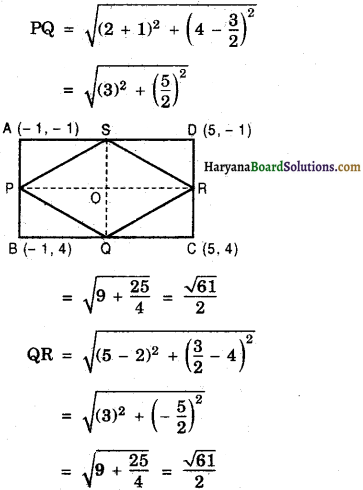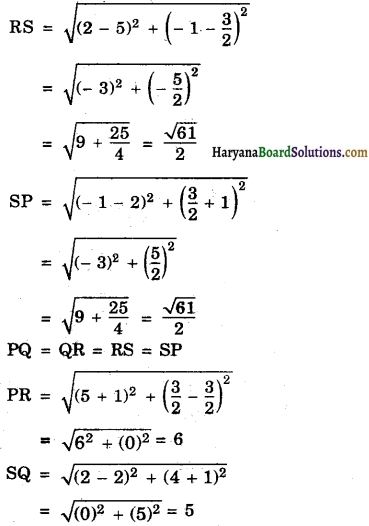Thus PQ = QR = RS = SP, but diagonal PR ≠ diagonal SQ.
Hence quadrilateral PQRS is a rhombus.